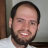sicp-solutions

In an ideal world, this page will grow and eventually list all solutions to sicp. Suggested guidelines:

• prefix the solution number with sicp-ex-, so the pages will list nicely in the wiki
• only use concepts explained up to that point; e.g. don't use cons, car, cdr etc in section 1 solutions
• solutions should be in Scheme, not Common Lisp (this is a Scheme wiki, after all)

Solutions

Chapter 5. Computing with Register Machines

5.2 A Register Machine SimulatorLenatis

Hey guys i have also put part of my sicp solution on blogger http://www.lenatis.net .I'd like to hear from you,my email is lenatis@gmail,comments and contact are welcome!!

Ivan Veselov

There is a wiki dedicated to SICP with solutions available in Scheme, Haskell and Oberon. Also discussions of solutions are present. http://sicp.org.ua

r2q2

Some solutions I have completed

``` ;;This program computes the cube root of a number. It also is in block structure

(define (exercise-1.8 x)
(define (good-enough? guess)
(< (abs (- (cube guess) x)) 0.0001))
(define (cubert-iter guess)
(if (good-enough? guess)
guess
(cubert-iter (improve guess))))
(define (improve guess)
(/ (+ (/ x (* guess guess)) (* 2 guess)) 3))
(define (cube y) (* y y y))
(cubert-iter 1.0))
```Michael-Micek

You might try this again after completing exercise 1.7; it has the same problems the sqrt defined in the text has.

``` ;;http://mitpress.mit.edu/sicp/full-text/book/book-Z-H-11.html#%_thm_1.11
;;Note f(n) is in recursive form
(define (f n)
(cond ((< n 3) n)
((>= n 3)
(+
(f (- n 1))
(* 2 (f (- n 2)))
(* 3 (f (- n 3)))))))
;;Testing
(f 0)
(f 5)
(f 10)

```Ken-Dyck

In the same vein, I've been posting SICP solutions on my blog: http://www.kendyck.com/solutions-to-sicp/

Ivan

I have solved as well most of the exercises. I tried to do them in a systematic and explanatory way so others can benefit from using them. https://github.com/ivanjovanovic/sicp

Eliben

I began posting my "readings" and exercise solutions of SICP in my blog: http://eli.thegreenplace.net/category/programming/lisp/sicp The examples and solutions are rewritten in Common Lisp instead of Scheme.

Brit Butler

I've also begun blogging my SICP solutions in scheme and providing a little commentary. http://redlinernotes.com/blog/?cat=39

flamingo

My attempt to complete SICP book here https://github.com/flaming0

voom4000

I started to post raw solutions (sometimes with debug output) here http://voom4000.wordpress.com/ I also publish interesting things from the book and video, e.g. iterative tower of Hanoi, analysis of counting change, etc. If you tried to solve some exercises and want to discuss some solutions or details, welcome!

AbdulFattaah

My solutions to the SICP book are available here: https://github.com/abdulapopoola/SICPBook

poly

Welcome to my github: https://github.com/collins562/SICP-solutions

postboy

Just another repository with solutions: https://github.com/postboy/sicp-homework